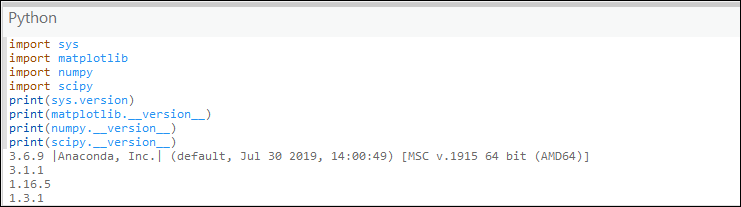Continue in the app
Be The First To Get Support Updates

# 常见问题解答：ArcGIS 中使用的 Python 是什么版本？

## 答案

`注： 建议不要安装用于 ArcGIS Desktop 的独立版本 Python。 使用不同版本的 Python 会导致兼容性问题和根文件夹问题。 不支持替换 ArcGIS Server 随附的 Python 版本。`

ArcGIS Pro

`import sys import matplotlib import numpy import scipy print(sys.version) print(matplotlib.__version__) print(numpy.__version__) print(scipy.__version__)``注： 在 ArcGIS Pro 1.0 到 1.2 版本中，如果在 ArcGIS Pro 之外使用 Python，则需要安装 Python for ArcGIS Pro。 在版本 1.3 及更高版本中，无需执行此操作。`

ArcGIS Desktop

 ArcGIS Desktop 版本 Python 和 NumPy 版本 10.8.1 Python 2.7.18 和 NumPy 1.9.3 10.8 Python 2.7.16 和 NumPy 1.9.3 10.7.1 Python 2.7.16 和 NumPy 1.9.3 10.7 Python 2.7.15 和 NumPy 1.9.3 10.6.1 Python 2.7.14 和 NumPy 1.9.3 10.6 Python 2.7.14 和 NumPy 1.9.3 10.5.1 Python 2.7.13 和 NumPy 1.9.3 10.5 Python 2.7.12 和 NumPy 1.9.3 10.4.x Python 2.7.10 和 NumPy 1.9.2 10.3.x Python 2.7.8 和 NumPy 1.7.1 10.2.1 Python 2.7.5 和 NumPy 1.7.1 10.2.2 Python 2.7.5 和 NumPy 1.7.1 10.2 Python 2.7.3 和 NumPy 1.6.1 10.1 Python 2.7.2 和 NumPy 1.6.1 10.0 Python 2.6.5 和 NumPy 1.3.0

ArcGIS Enterprise

ArcGIS Enterprise 还随附 Python。 在最新版本中，将提供 Python 2.x 和 Python 3.x 运行时。

 ArcGIS Enterprise 版本 Python、NumPy 和 Matplotlib 版本 10.9 Python 2.7.18、NumPy 1.9.3 和 matplotlib 1.5.2 10.9 Python 3.7.9、NumPy 1.19.1 和 matplotlib 3.3.1 10.8.1 Python 2.7.18、NumPy 1.9.3 和 matplotlib 1.5.2 10.8.1 Python 3.6.10、NumPy 1.18.1 和 matplotlib 3.2.1 10.8 Python 2.7.16、NumPy 1.9.3 和 matplotlib 1.5.2 10.8 Python 3.6.9、NumPy 1.16.5 和 matplotlib 3.1.1 10.7.1 Python 2.7.16、NumPy 1.9.3 和 matplotlib 1.5.2 10.7.1 Python 3.6.8、NumPy 1.16.2 和 matplotlib 3.0.3 10.7 Python 2.7.15、NumPy 1.9.3 和 matplotlib 1.5.2 10.7 Python 3.6.6、NumPy 1.15.1 和 matplotlib 2.2.3 10.6.1 Python 2.7.14、NumPy 1.9.3 和 matplotlib 1.5.2 10.6.1 Python 3.6.5、NumPy 1.14.2 和 matplotlib 2.2.2 10.6 Python 2.7.14、NumPy 1.9.3 和 matplotlib 1.5.2 10.6 Python 3.6.2 10.5.1 Python 2.7.13、NumPy 1.9.3 和 matplotlib 1.5.2 10.5.1 Python 3.5.3 10.5 Python 2.7.12、NumPy 1.9.3 和 matplotlib 1.5.2 10.5 Python 3.5.2 10.4 和 10.4.1 Python 2.7.10、NumPy 1.9.2 和 matplotlib 1.4.3 10.4 和 10.4.1 Python 3.4.1 10.3.x Python 2.7.8、NumPy 1.7.1 和 matplotlib 1.3.0 10.2.1 和 10.2.2 Python 2.7.5 和 NumPy 1.7.1 10.2 Python 2.7.3 和 NumPy 1.6.1 10.1 Python 2.7.2 和 NumPy 1.6.1 10.0 Python 2.6.5 和 NumPy 1.3.0

ArcGIS Notebook Server

ArcGIS Notebook Server 中的每个 notebook 运行时都打包了精确的 Python 库列表，包括每个库的特定版本。 如果您需要一个默认情况下不处于任一运行时的库，您可以扩展 notebook 运行时以包括该库。 有关每个默认运行时中打包的 Python 库的完整列表，请参阅 ArcGIS Notebook Server：可用 Python 库